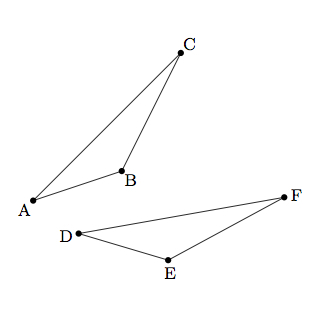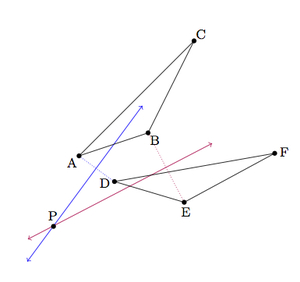# Identifying Rotations

Alignments to Content Standards: G-CO.A.4

Below is triangle $ABC$ and a rotated image triangle $DEF$.1. Explain how to identify the center of rotation.
2. Once you have found the center, how do you find the angle of rotation?

## IM Commentary

The purpose of this task is to use the definition of rotations in order to find the center and angle of rotation given a triangle and its image under a rotation. This will help students gain a solid grasp of the definition rotations while also giving them a chance to use this definition in solving problems. The statement of the task does not provide any guidance and the teacher may wish to draw some pictures or ask questions in order to orient students towards considering the perpendicular bisectors of the segments joining vertices of $\triangle ABC$ to their rotated images in $\triangle DEF$.

Teachers may wish to use technology to complement this task, allowing students to explore rotations with different centers to see their impact on triangles or other figures.

## Solution

1. Suppose we call $P$ the center of the rotation. We know that $|AP| = |DP|$, $|BP| = |EP|$, and $|CP| = |FP|$ because rotations preserve distances. Since $P$ is equidistant from $A$ and $D$ it lies on the perpendicular bisectors of $\overline{AD}$ and $P$ similarly lies on the perpendicular bisector of $\overline{BE}$ and $\overline{CF}$. This provides us with a means of identifying $P$ as long as these perpendicular bisectors are not all the same line:The picture above shows an example of the case where the perpendicular bisector of $\overline{AD}$ meets the perpendicular bisector of $\overline{BD}$ in a single point $P$. In this case, $P$ is the center of the rotation and the angle of rotation is $m(\angle APD)$ which is the same as $m(\angle BPE)$ and $m(\angle CPF)$.

Next we examine the case where the perpendicular bisector of $\overline{AD}$ is the same as the perpendicular bisector of $\overline{BE}$. We know, moreover that $\overrightarrow{PA}$ and $\overrightarrow{PB}$ make the same angle (namely half of the angle of rotation) with the ray of the perpendicular bisector of $\overline{AD}$ and $\overline{BC}$ which is interior to $\angle APD$ and $\angle BPD$. This means that $A$, $B$, and $P$ are collinear. Since $A, B, C,$ and $P$ can not all be collinear (otherwise $ABC$ would not be a triangle), we can then use the perpendicular bisectors of $\overline{AD}$ and $\overline{CF}$ which will intersect in the center of rotation $P$.

2. We answered this in the work on part (a): the angle of rotation can be taken as $m(\angle APD)$, $m(\angle BPE)$, or $m(\angle CPF)$, all of which are equal. This comes from the definition of a rotation: if $r$ denotes a rotation about $P$ by $d$ degrees, and $x \neq P$ then $r(x)$ is the point in the plane with $|xP| = |r(x)P|$ and $m(\angle xPr(x)) = d$.